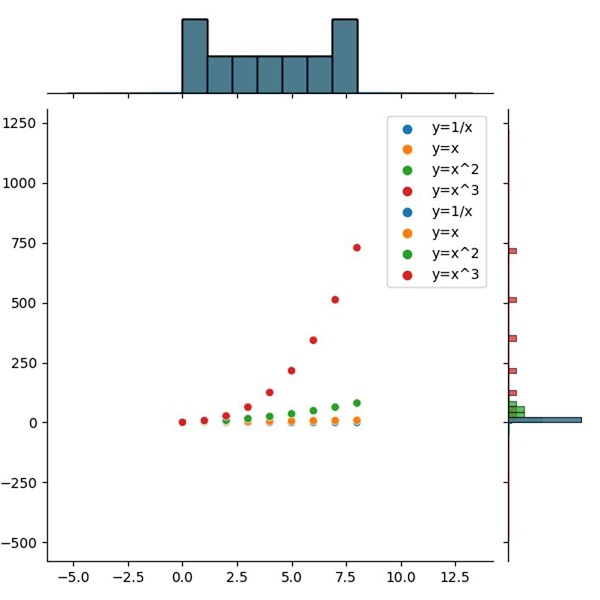# How to plot multiple Seaborn Jointplot in Subplot using Matplotlib?

To plot multiple Seaborn jointplot, we can use the jointplot() method.

## Steps

• Add a subplot to the current figure.

• Create a dictionary, with some keys.

• Create a dataframe using Pandas.

• Make a jointplot using the jointplot() method.

• To plot the curves, use the plot() method.

• To display the figure, use the show() method.

## Example

from matplotlib import pyplot as plt
import pandas as pd
import seaborn as sns
plt.rcParams["figure.figsize"] = [7.50, 3.50]
plt.rcParams["figure.autolayout"] = True
plt.subplot()
d = {
'y=1/x': [1 / i for i in range(1, 10)],
'y=x': [i for i in range(1, 10)],
'y=x^2': [i * i for i in range(1, 10)],
'y=x^3': [i * i * i for i in range(1, 10)]
}
df = pd.DataFrame(d)
jg = sns.jointplot(data=df)
jg.plot(sns.scatterplot, sns.histplot)
plt.show()

## Output